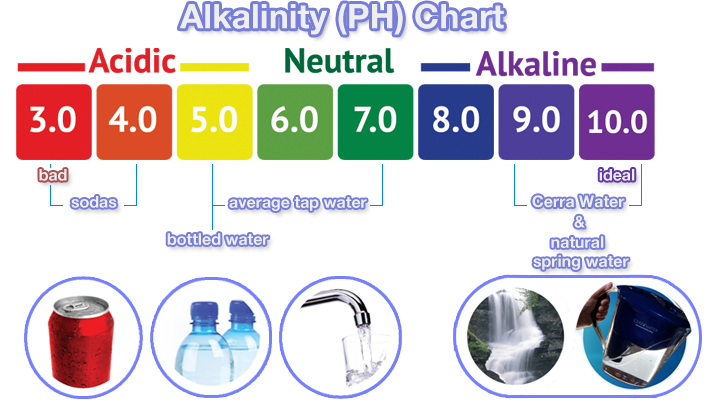# Determine The Alkalinity Of Given Water Sample Exprement Presentation

Introduction
Alkalinity is the measure of a water sample's capacity to resist changes in pH when an acid is added.

It is an important parameter to assess water quality and its suitability for various applications.

In this experiment, we will determine the alkalinity of a given water sample using a simple titration method.
Image Source1

Materials and Procedure
Materials: - Water sample - Burette - Titration flask - Phenolphthalein indicator - Standardized hydrochloric acid (HCl) - Graduated cylinder

Procedure: 1. Collect a representative water sample and measure its volume accurately. 2. Add a few drops of phenolphthalein indicator to the titration flask. 3. Fill the burette with standardized HCl solution. 4. Slowly add HCl solution drop by drop to the water sample while stirring. 5. Observe the color change in the solution. The color will disappear when alkalinity is neutralized.2

Results and Calculation
Results: - Record the volume of HCl solution used to neutralize the alkalinity in the water sample. - Calculate the alkalinity using the formula: Alkalinity (mg/ L) = (Volume of HCl used × Normality of HCl × 50000) / Volume of water sample.

Example calculation: - Volume of HCl used: 15 mL - Normality of HCl: 0.1 N - Volume of water sample: 100 mL - Alkalinity (mg/ L) = (15 × 0.1 × 50000) / 100 = 750 mg/ L3

Conclusion and Significance
Conclusion: - The alkalinity of the given water sample was determined to be 750 mg/ L using the titration method. - This indicates the water sample has a relatively high alkalinity level.

Significance: - Knowledge of alkalinity is crucial for various applications, such as drinking water treatment, aquatic ecosystems, and industrial processes. - Monitoring alkalinity helps understand the buffering capacity of water and its ability to resist pH changes. - Regular testing of alkalinity ensures the maintenance of water quality standards and prevents adverse effects on the environment and human health.